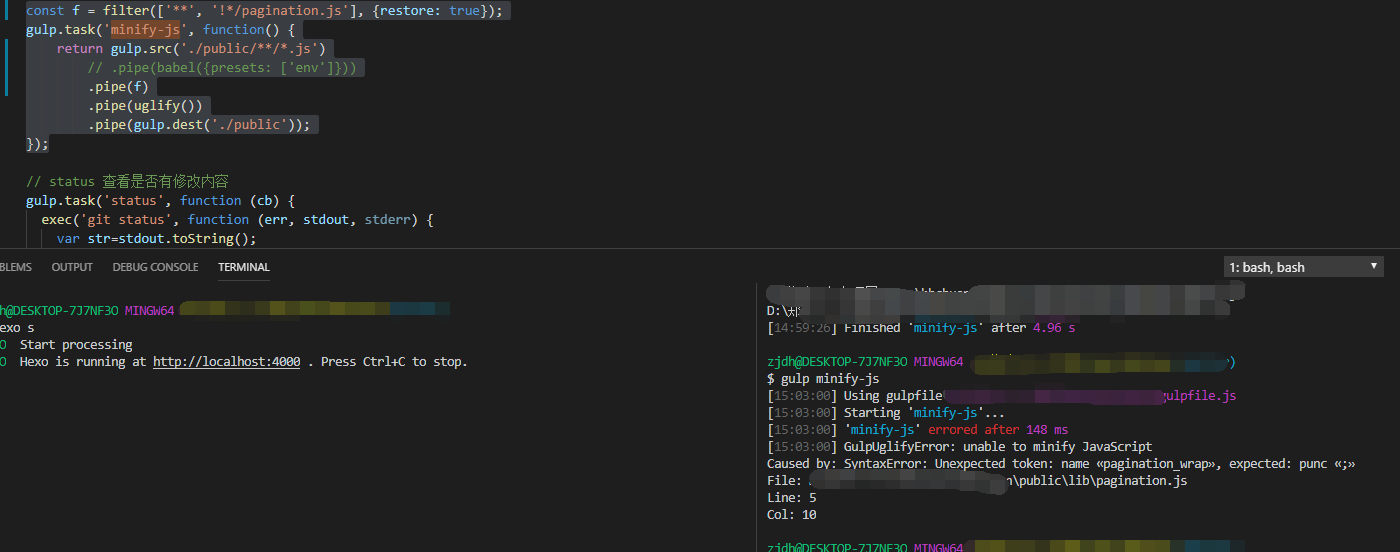{{ message }}

# 精通 gulp 常用插件的功能和用法 #2

Open
opened this issue Mar 9, 2017 · 23 comments
Open

# 精通 gulp 常用插件的功能和用法#2

opened this issue Mar 9, 2017 · 23 comments
Labels

###lin-xin commented Mar 9, 2017 • edited

gulp的官方定义非常简洁： 基于文件流的构建系统 。通过代码优于配置的策略，Gulp 让简单的任务简单，复杂的任务可管理。利用 Node.js 流的威力，你可以快速构建项目并减少频繁的 IO 操作。Gulp 严格的插件指南确保插件如你期望的那样简洁高质得工作。

## 匹配符 *、**、！、{}

```src('./js/*.js')               // * 匹配js文件夹下所有.js格式的文件
src('./js/**/*.js')            // ** 匹配js文件夹的0个或多个子文件夹
src(['./js/*.js','!./js/index.js'])    // ! 匹配除了index.js之外的所有js文件
src('./js/**/{omui,common}.js')        // {} 匹配{}里的文件名```

## 文件操作

### del (替代gulp-clean)

```const del = require('del');

del('./dist');                      // 删除整个dist文件夹```

### gulp-rename

```const { src, dest } = require('gulp');
const rename = require("gulp-rename");

const renameTask = cb => {
src('./hello.txt')
.pipe(rename('gb/goodbye.md'))    // 直接修改文件名和路径
.pipe(dest('./dist'));
cb();
}
const optionTask = cb => {
src('./hello.txt')
.pipe(rename({
dirname: "text",                // 路径名
basename: "goodbye",            // 主文件名
prefix: "pre-",                 // 前缀
suffix: "-min",                 // 后缀
extname: ".html"                // 扩展名
}))
.pipe(dest('./dist'));
cb();
}```

### gulp-concat

```const { src, dest } = require('gulp');
const concat = require('gulp-concat');

const concatTask = cb => {
src('./js/*.js')
.pipe(concat('all.js'))         // 合并all.js文件
.pipe(dest('./dist'));
cb();
}```

### gulp-filter

```const { src, dest } = require('gulp');
const filter = require('gulp-filter');

const f = filter(['**', '!*/index.js']);
const fTask = cb => {
src('js/**/*.js')
.pipe(f)                        // 过滤掉index.js这个文件
.pipe(dest('dist'));
cb();
}

const f1 = filter(['**', '!*/index.js'], {restore: true});
const f1Task = cb => {
src('js/**/*.js')
.pipe(f1)                       // 过滤掉index.js这个文件
.pipe(uglify())                 // 对其他文件进行压缩
.pipe(f1.restore)               // 返回到未过滤执行的所有文件
.pipe(dest('dist'));       // 再对所有文件操作，包括index.js
cb();
}```

## 压缩

### gulp-uglify

```const { src, dest } = require('gulp');
const uglify = require("gulp-uglify");

const uglifypTask = cb => {
src('./hello.js')
.pipe(uglify())                 // 直接压缩hello.js
.pipe(dest('./dist'))
cb();
}

const optionpTask = cb => {
src('./hello.js')
.pipe(uglify({
mangle: true,               // 是否修改变量名，默认为 true
compress: true,             // 是否完全压缩，默认为 true
}))
.pipe(dest('./dist'))
cb();
}```

### gulp-csso

```const { src, dest } = require('gulp');
const csso = require('gulp-csso');

const cssopTask = cb => {
src('./css/*.css')
.pipe(csso())
.pipe(dest('./dist/css'))
cb();
}```

### gulp-html-minify

```const { src, dest } = require('gulp');
const htmlmini = require('gulp-htmlmin');

const minpTask = cb => {
src('index.html')
.pipe(htmlmini())
.pipe(dest('./dist'))
cb();
}```

### gulp-imagemin

```const { src, dest } = require('gulp');
const imagemin = require('gulp-imagemin');

const minpTask = cb => {
src('./img/*.{jpg,png,gif,ico}')
.pipe(imagemin())
.pipe(dest('./dist/img'))
cb();
}```

### gulp-zip

```const { src, dest } = require('gulp');
const zip = require('gulp-zip');

const zipTask = cb => {
src('./src/*')
.pipe(zip('all.zip'))                   // 压缩成all.zip文件
.pipe(dest('./dist'))
cb();
}```

## JS/CSS自动注入

### gulp-autoprefixer

```const { src, dest } = require('gulp');
const autoprefixer = require('gulp-autoprefixer');

const prefTask = cb => {
src('./css/*.css')
.pipe(autoprefixer())           // 直接添加前缀
.pipe(dest('dist'))
cb();
}
const optionTask = cb => {
src('./css/*.css')
.pipe(autoprefixer({
browsers: ['last 2 versions'],      // 浏览器版本
remove: true                        // 删除过时前缀，默认true
flexbox: true                       // 为flexbox属性添加前缀，默认true
}))
.pipe(dest('./dist'))
cb();
}```

### gulp-useref

```<!-- index.html -->
<!-- build:css /css/all.css -->
<!-- endbuild -->```
```// gulpfile.js
const { src, dest } = require('gulp');
const useref = require('gulp-useref');
const userefTask = cb => {
src('index.html')
.pipe(useref())
.pipe(dest('./dist'))
cb();
}```

`<link rel="stylesheet" href="css/all.css">  // 之前的两个<link>替换成一个了`

### gulp-rev

```const { src, dest } = require('gulp');
const rev = require('gulp-rev');

const revTask = cb => {
src('./css/*.css')
.pipe(rev())
.pipe(dest('./dist/css'))
cb();
}```

### gulp-rev-replace

```const { src, dest } = require('gulp');
const rev = require('gulp-rev');
const revReplace = require('gulp-rev-replace');
const useref = require('gulp-useref');

const revReplaceTask = cb => {
src('index.html')
.pipe(useref())                         // 替换HTML中引用的css和js
.pipe(rev())                            // 给css,js,html加上hash版本号
.pipe(revReplace())                     // 把引用的css和js替换成有版本号的名字
.pipe(dest('./dist'))
cb();
}```

### gulp-html-replace

```<!-- index.html -->

<!-- build:css -->                          // css是buildName,可以自己定义
<!-- endbuild -->```
```// gulpfile.js
const { src, dest } = require('gulp');
const htmlreplace = require('gulp-html-replace');

const replaceTask = cb => {
src('index.html')
.pipe(htmlreplace({
'css':'all.css'                     // css是index.html中定义的buildName
}))
.pipe(dest('./dist'))
cb();
}```

`    <link rel="stylesheet" href="all.css">      <!-- 之前的两个<link>替换成一个了 -->`

## 流控制

### gulp-if

```const { src, dest } = require('gulp');
const gulpif = require('gulp-if');
const uglify = require('gulp-uglify');
const concat = require('gulp-concat');
const condition = true;

const ifTask = cb => {
src('./js/*.js')
.pipe(gulpif(condition, uglify(), concat('all.js')))  // condition为true时执行uglify(), else 执行concat('all.js')
.pipe(dest('./dist/'));
cb();
}```

## 工具

```// package.json

"devDependencies": {
"gulp": "^3.9.1",
"gulp-concat": "^2.6.1",
"gulp-rename": "^1.2.2",
"gulp-uglify": "^2.0.1"
}

// gulpfile.js
const { src, dest } = require('gulp');
const \$ = require('gulp-load-plugins')();     // \$ 是一个对象,加载了依赖里的插件

const minTask = cb => {
src('./**/*.js')
.pipe(\$.concat('all.js'))               // 使用插件就可以用\$.PluginsName()
.pipe(\$.uglify())
.pipe(\$.rename('all.min.js'))
.pipe(dest('./dist'))
cb();
}```

### gulp-sass

```const { src, dest, watch } = require('gulp');
const sass = require('gulp-sass');

const sassTask = cb => {
src('./sass/**/*.scss')
.pipe(sass({
outputStyle: 'compressed'           // 配置输出方式,默认为nested
}))
.pipe(dest('./dist/css'));
cb();
}

### gulp-babel

```const { src, dest } = require('gulp');
const babel = require('gulp-babel');

const jsTask = cb => {
src('./js/index.js')
.pipe(babel({
presets: ['@babel/env'],
sourceType: 'script'
}))
.pipe(dest('./dist'))
cb();
}```

###jweboy commented Apr 26, 2017

 Mark
1 similar comment

 Mark

###yang123guo commented Jul 14, 2017

 mark an use it

###Mengkzhaoyun commented Jul 28, 2017

 Mark
1 similar comment

 Mark

 M

 M

 M

 Mark

 M

 Mark

 mark

 m

###wuyf123 commented Jun 22, 2018

 mark
1 similar comment

 mark

 Mark!

###unclemeric commented Jul 27, 2018

 mark
1 similar comment

 mark

###zhangji1992 commented Aug 20, 2018

 thx.It helps a lot.

 thx

###bibichuan commented Aug 8, 2019

 `const f = filter(['**', '!*/pagination.js'], {restore: true}); gulp.task('minify-js', function() { return gulp.src('./public/**/*.js') // .pipe(babel({presets: ['env']})) .pipe(f) .pipe(uglify()) .pipe(gulp.dest('./public')); });`我想把public文件夹中的pagination.js过滤掉，但是这样写，pagination.js还是会被处理和压缩，这是什么原因呢？和windows系统有关系吗？###touxing commented Sep 11, 2019

 `const f = filter(['**', '!*/pagination.js'], {restore: true}); gulp.task('minify-js', function() { return gulp.src('./public/**/*.js') // .pipe(babel({presets: ['env']})) .pipe(f) .pipe(uglify()) .pipe(gulp.dest('./public')); });`我想把public文件夹中的pagination.js过滤掉，但是这样写，pagination.js还是会被处理和压缩，这是什么原因呢？和windows系统有关系吗？读取js和压缩后的js文件放在同一个目录可能会存在问题 你可以这样写 ```gulp.task('minify-js', function() { return gulp.src(['./public/**/*.js', '!./public/**/pagination.js']) .pipe(uglify()) .pipe(gulp.dest('./otherFolder')) })```

###bibichuan commented Sep 11, 2019

 谢谢 @touxing